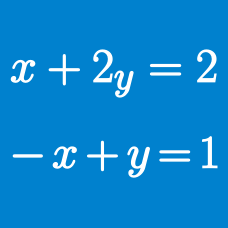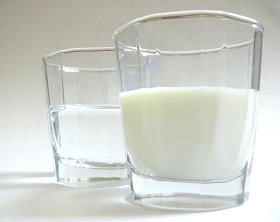Algebra

System of Equations: Level 3 ChallengesThere are two containers named $A$ and $B$. Container $A$ has milk in it, container $B$ has water (same amount as of milk in container $A$) in it. Some amount of milk is transferred from container $A$ to container $B$ and then , the same amount is transferred from the container $B$ to the container $A$.

Let container $A$ has $x$ amount of water in it and container $B$ has $y$ amount of milk in it.

Which one option is correct?

Note : Finally the amount in both the containers is same as it was initially.

Find the number of real solutions $(x,y,z)$ of

$\begin{cases} (x+y)^{3}=z \\ (y+z)^{3}=x \\ (z+x)^{3}=y \end{cases}$

What is the largest integer $n \leq 1000$, such that there exist 2 non-negative integers $(a, b)$ satisfying

$n = \frac{ a^2 + b^2 } { ab - 1 } ?$

Hint: $(a,b) = (0,0)$ gives us $\frac{ 0^2 + 0^2 } { 0 \times 0 - 1 } = 0$, so the answer is at least $0 .$

$\begin{cases} (1+x)(1+x^2)(1+x^4) = 1+y^7 \\ (1+y)(1+y^2)(1+y^4) = 1+x^7 \end{cases}$

How many ordered pairs of real numbers $(x, y)$ satisfy the above equations?

$\left\{ \begin{array}{l} \frac{{bcdef}}{a} = 4\\ \frac{{acdef}}{b} = 9\\ \frac{{abdef}}{c} = 16\\ \frac{{abcef}}{d} = \frac{1}{4}\\ \frac{{abcdf}}{e} = \frac{1}{9}\\ \frac{{abcde}}{f} = \frac{1}{{16}} \end{array} \right.$ Positive numbers $a,b,c,d,e,f$ satisfy the formulas above. Find the value of $(a+ c + e) - (b + d + f)$

×